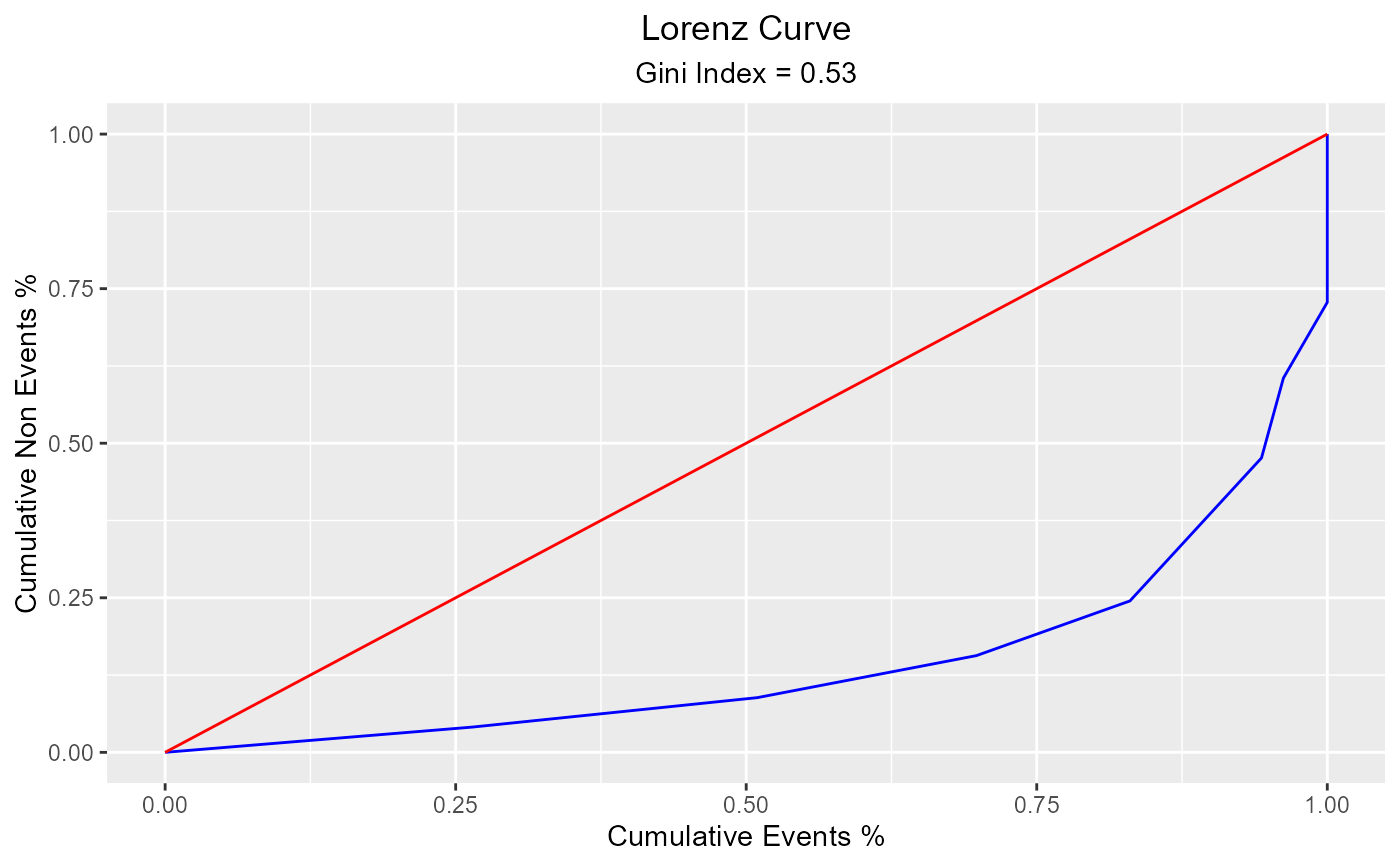Lorenz curve is a visual representation of inequality. It is used to measure the discriminatory power of the predictive model.

blr_lorenz_curve(
model,
data = NULL,
title = "Lorenz Curve",
xaxis_title = "Cumulative Events %",
yaxis_title = "Cumulative Non Events %",
diag_line_col = "red",
lorenz_curve_col = "blue",
print_plot = TRUE
)

## Arguments

model An object of class glm. A tibble or data.frame. Plot title. X axis title. Y axis title. Diagonal line color. Color of the lorenz curve. logical; if TRUE, prints the plot else returns a plot object.

Other model validation techniques: blr_confusion_matrix(), blr_decile_capture_rate(), blr_decile_lift_chart(), blr_gains_table(), blr_gini_index(), blr_ks_chart(), blr_roc_curve(), blr_test_hosmer_lemeshow()
model <- glm(honcomp ~ female + read + science, data = hsb2,